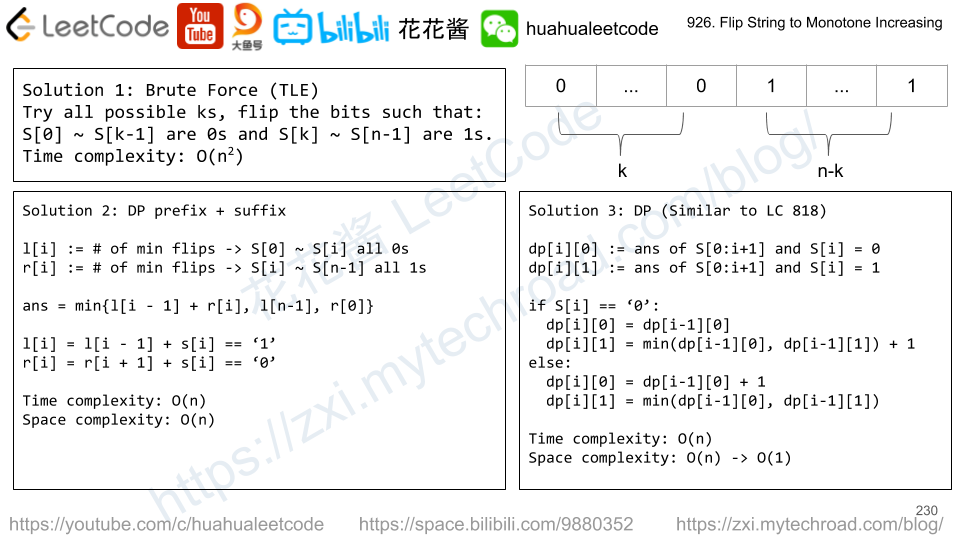Press "Enter" to skip to content

# Problem

Given a square array of integers A, we want the minimum sum of a falling path through A.

A falling path starts at any element in the first row, and chooses one element from each row.  The next row’s choice must be in a column that is different from the previous row’s column by at most one.

Example 1:

Input: [[1,2,3],[4,5,6],[7,8,9]]
Output: 12
Explanation:
The possible falling paths are:

• [1,4,7], [1,4,8], [1,5,7], [1,5,8], [1,5,9]
• [2,4,7], [2,4,8], [2,5,7], [2,5,8], [2,5,9], [2,6,8], [2,6,9]
• [3,5,7], [3,5,8], [3,5,9], [3,6,8], [3,6,9]

The falling path with the smallest sum is [1,4,7], so the answer is 12.

Note:

1. 1 <= A.length == A.length <= 100
2. -100 <= A[i][j] <= 100

# Solution: DP

Time complexity: O(mn)

Space complexity: O(mn)

## C++/in place

Every email consists of a local name and a domain name, separated by the @ sign.

For example, in alice@leetcode.comalice is the local name, and leetcode.com is the domain name.

Besides lowercase letters, these emails may contain '.'s or '+'s.

If you add periods ('.') between some characters in the local name part of an email address, mail sent there will be forwarded to the same address without dots in the local name.  For example, "alice.z@leetcode.com" and "alicez@leetcode.com" forward to the same email address.  (Note that this rule does not apply for domain names.)

If you add a plus ('+') in the local name, everything after the first plus sign will be ignored. This allows certain emails to be filtered, for example m.y+name@email.com will be forwarded to my@email.com.  (Again, this rule does not apply for domain names.)

It is possible to use both of these rules at the same time.

Given a list of emails, we send one email to each address in the list.  How many different addresses actually receive mails?

Example 1:

Input: ["test.email+alex@leetcode.com","test.e.mail+bob.cathy@leetcode.com","testemail+david@lee.tcode.com"]
Output: 2
Explanation: "testemail@leetcode.com" and "testemail@lee.tcode.com" actually receive mails


Note:

• 1 <= emails[i].length <= 100
• 1 <= emails.length <= 100
• Each emails[i] contains exactly one '@' character.

# Solution:

Time complexity: O(n*l)
Space complexity: O(n*l)

# Problem

Given an array A of 0s and 1s, divide the array into 3 non-empty parts such that all of these parts represent the same binary value.

If it is possible, return any [i, j] with i+1 < j, such that:

• A, A, ..., A[i] is the first part;
• A[i+1], A[i+2], ..., A[j-1] is the second part, and
• A[j], A[j+1], ..., A[A.length - 1] is the third part.
• All three parts have equal binary value.

If it is not possible, return [-1, -1].

Note that the entire part is used when considering what binary value it represents.  For example, [1,1,0] represents 6 in decimal, not 3.  Also, leading zeros are allowed, so [0,1,1] and [1,1] represent the same value.

Example 1:

Input: [1,0,1,0,1]
Output: [0,3]


Example 2:

Input: [1,1,0,1,1]
Output: [-1,-1]

Note:

1. 3 <= A.length <= 30000
2. A[i] == 0 or A[i] == 1

# Solution:

each part should have the same number of 1 s.

Find the suffix (without leading os) of the last part which should have 1/3 of the total ones.

Time complexity: O(n^2) in theory but close to O(n) in practice

Space complexity: O(n)

# Problem

A string of '0's and '1's is monotone increasing if it consists of some number of '0's (possibly 0), followed by some number of '1's (also possibly 0.)

We are given a string S of '0's and '1's, and we may flip any '0' to a '1' or a '1' to a '0'.

Return the minimum number of flips to make S monotone increasing.

Example 1:

Input: "00110"
Output: 1
Explanation: We flip the last digit to get 00111.


Example 2:

Input: "010110"
Output: 2
Explanation: We flip to get 011111, or alternatively 000111.


Example 3:

Input: "00011000"
Output: 2
Explanation: We flip to get 00000000.


Note:

1. 1 <= S.length <= 20000
2. S only consists of '0' and '1' characters.

# Solution: DPl[i] := number of flips to make S ~ S[i] become all 0s.

r[i] := number of flips to make S[i] ~ S[n – 1] become all 1s.

Try all possible break point, S ~ S[i – 1] are all 0s and S[i] ~ S[n-1] are all 1s.

ans = min{l[i – 1] + r[i]}

Time complexity: O(n)

Space complexity: O(n)

# Problem

Your friend is typing his name into a keyboard.  Sometimes, when typing a character c, the key might get long pressed, and the character will be typed 1 or more times.

You examine the typed characters of the keyboard.  Return True if it is possible that it was your friends name, with some characters (possibly none) being long pressed.

Example 1:

Input: name = "alex", typed = "aaleex"
Output: true
Explanation: 'a' and 'e' in 'alex' were long pressed.


Example 2:

Input: name = "saeed", typed = "ssaaedd"
Output: false
Explanation: 'e' must have been pressed twice, but it wasn't in the typed output.


Example 3:

Input: name = "leelee", typed = "lleeelee"
Output: true


Example 4:

Input: name = "laiden", typed = "laiden"
Output: true
Explanation: It's not necessary to long press any character.


Note:

1. name.length <= 1000
2. typed.length <= 1000
3. The characters of name and typed are lowercase letters.

# Solution: Two Pointers

Time complexity: O(n)

Space complexity: O(1)

## C++ / Iterator

Mission News Theme by Compete Themes.